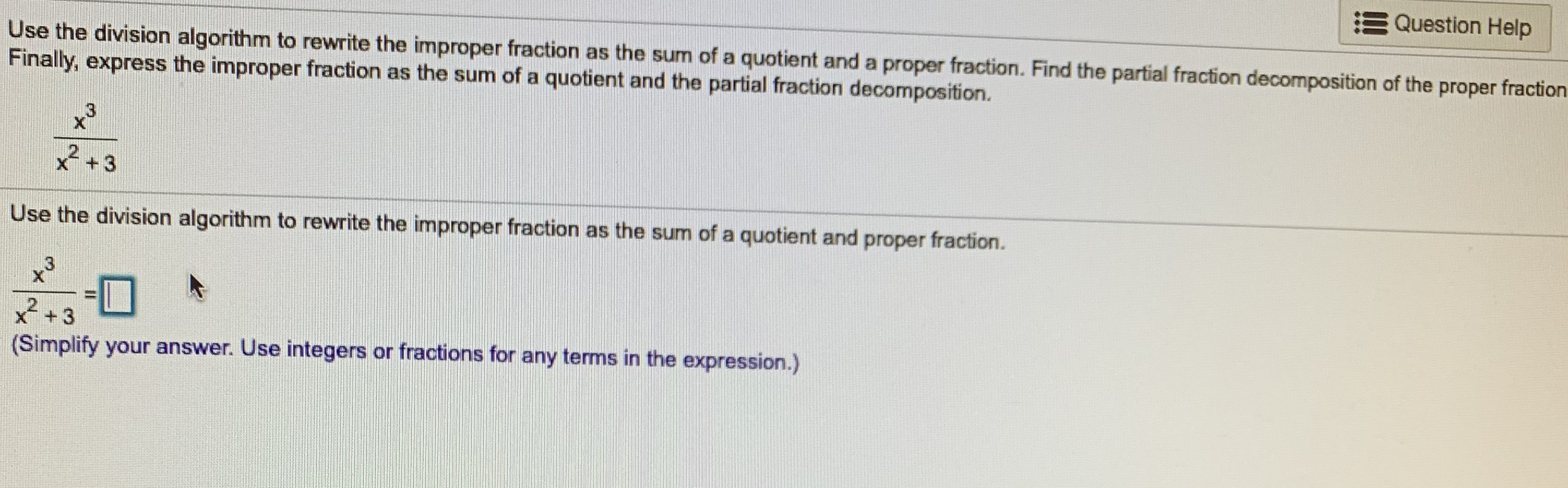# Question HelpUse the division algorithm to rewrite the improper fraction as the sum of a quotient and a proper fraction. Find the partial fraction decomposition of the proper fractionFinally, express the improper fraction as the sum of a quotient and the partial fraction decomposition.2+3Use the division algorithm to rewrite the improper fraction as the sum of a quotient and proper fraction.C3XT3(Simplify your answer. Use integers or fractions for any terms in the expression.)

Question
6 viewshelp_outlineImage TranscriptioncloseQuestion Help Use the division algorithm to rewrite the improper fraction as the sum of a quotient and a proper fraction. Find the partial fraction decomposition of the proper fraction Finally, express the improper fraction as the sum of a quotient and the partial fraction decomposition. 2+3 Use the division algorithm to rewrite the improper fraction as the sum of a quotient and proper fraction. C3 XT 3 (Simplify your answer. Use integers or fractions for any terms in the expression.) fullscreen
check_circle

Step 1

Given that, the improper fraction is

Step 2

By division algorithm the improper fraction is written as the sum of quotient and proper fraction as,

Step 3

Simplify the above ...

### Want to see the full answer?

See Solution

#### Want to see this answer and more?

Solutions are written by subject experts who are available 24/7. Questions are typically answered within 1 hour.*

See Solution
*Response times may vary by subject and question.
Tagged in

### Calculus